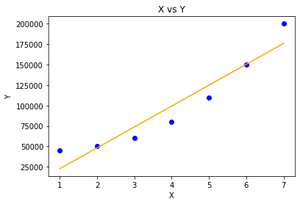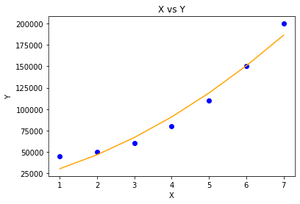Related Articles

# Polynomial Regression ( From Scratch using Python )

• Difficulty Level : Medium
• Last Updated : 22 Jun, 2021

#### Prerequisites

1. Linear Regression

#### Introduction

Linear Regression finds the correlation between the dependent variable ( or target variable ) and independent variables ( or features ). In short, it is a linear model to fit the data linearly. But it fails to fit and catch the pattern in non-linear data.

Let’s first apply Linear Regression on non-linear data to understand the need for Polynomial Regression. The Linear Regression model used in this article is imported from sklearn. You can refer to the separate article for the implementation of the Linear Regression model from scratch.

## Python3

 `# Importing libraries` `import` `numpy as np` `import` `pandas as pd` `from` `sklearn.model_selection ``import` `train_test_split` `import` `matplotlib.pyplot as plt` `from` `sklearn.linear_model ``import` `LinearRegression` `# driver code` `def` `main() :``    ` `    ``# Create dataset``    ` `    ``X ``=` `np.array( [ [``1``], [``2``], [``3``], [``4``], [``5``], [``6``], [``7``] ] )``    ` `    ``Y ``=` `np.array( [ ``45000``, ``50000``, ``60000``, ``80000``, ``110000``, ``150000``, ``200000` `] )``    ` `    ``# Model training``    ` `    ``model ``=` `LinearRegression()` `    ``model.fit( X, Y )``    ` `    ``# Prediction` `    ``Y_pred ``=` `model.predict( X )``    ` `    ``# Visualization``    ` `    ``plt.scatter( X, Y, color ``=` `'blue'` `)``    ` `    ``plt.plot( X, Y_pred, color ``=` `'orange'` `)``    ` `    ``plt.title( ``'X vs Y'` `)``    ` `    ``plt.xlabel( ``'X'` `)``    ` `    ``plt.ylabel( ``'Y'` `)``    ` `    ``plt.show()``    ` `    ` `if` `__name__ ``=``=` `"__main__"` `:``    ` `    ``main()`

#### Output :Visualization

As shown in the output visualization, Linear Regression even failed to fit the training data well ( or failed to decode the pattern in the Y with respect to X ). Because its hypothetical function is linear in nature and Y is a non-linear function of X in the data.

```For univariate linear regression :

h( x ) = w * x

here,  x is the feature vector.
and w is the weight vector.```

This problem is also called as underfitting. To overcome the underfitting, we introduce new features vectors just by adding power to the original feature vector.

```For univariate polynomial regression :

h( x ) = w1x + w2x2  + .... + wnxn

here, w is the weight vector.
where x2  is the derived feature from x. ```

After transforming the original X into their higher degree terms, it will make our hypothetical function able to fit the non-linear data. Here is the implementation of the Polynomial Regression model from scratch and validation of the model on a dummy dataset.

## Python

 `# Importing libraries` `import` `numpy as np` `import` `math` `import` `matplotlib.pyplot as plt` `# Univariate Polynomial Regression` `class` `PolynomailRegression() :``    ` `    ``def` `__init__( ``self``, degree, learning_rate, iterations ) :``        ` `        ``self``.degree ``=` `degree``        ` `        ``self``.learning_rate ``=` `learning_rate``        ` `        ``self``.iterations ``=` `iterations``        ` `    ``# function to transform X``    ` `    ``def` `transform( ``self``, X ) :``        ` `        ``# initialize X_transform``        ` `        ``X_transform ``=` `np.ones( ( ``self``.m, ``1` `) )``        ` `        ``j ``=` `0``    ` `        ``for` `j ``in` `range``( ``self``.degree ``+` `1` `) :``            ` `            ``if` `j !``=` `0` `:``                ` `                ``x_pow ``=` `np.power( X, j )``                ` `                ``# append x_pow to X_transform``                ` `                ``X_transform ``=` `np.append( X_transform, x_pow.reshape( ``-``1``, ``1` `), axis ``=` `1` `)` `        ``return` `X_transform  ``    ` `    ``# function to normalize X_transform``    ` `    ``def` `normalize( ``self``, X ) :``        ` `        ``X[:, ``1``:] ``=` `( X[:, ``1``:] ``-` `np.mean( X[:, ``1``:], axis ``=` `0` `) ) ``/` `np.std( X[:, ``1``:], axis ``=` `0` `)``        ` `        ``return` `X``        ` `    ``# model training``    ` `    ``def` `fit( ``self``, X, Y ) :``        ` `        ``self``.X ``=` `X``    ` `        ``self``.Y ``=` `Y``    ` `        ``self``.m, ``self``.n ``=` `self``.X.shape``    ` `        ``# weight initialization``    ` `        ``self``.W ``=` `np.zeros( ``self``.degree ``+` `1` `)``        ` `        ``# transform X for polynomial  h( x ) = w0 * x^0 + w1 * x^1 + w2 * x^2 + ........+ wn * x^n``        ` `        ``X_transform ``=` `self``.transform( ``self``.X )``        ` `        ``# normalize X_transform``        ` `        ``X_normalize ``=` `self``.normalize( X_transform )``                ` `        ``# gradient descent learning``    ` `        ``for` `i ``in` `range``( ``self``.iterations ) :``            ` `            ``h ``=` `self``.predict( ``self``.X )``        ` `            ``error ``=` `h ``-` `self``.Y``            ` `            ``# update weights``        ` `            ``self``.W ``=` `self``.W ``-` `self``.learning_rate ``*` `( ``1` `/` `self``.m ) ``*` `np.dot( X_normalize.T, error )``        ` `        ``return` `self``    ` `    ``# predict``    ` `    ``def` `predict( ``self``, X ) :``     ` `        ``# transform X for polynomial  h( x ) = w0 * x^0 + w1 * x^1 + w2 * x^2 + ........+ wn * x^n``        ` `        ``X_transform ``=` `self``.transform( X )``        ` `        ``X_normalize ``=` `self``.normalize( X_transform )``        ` `        ``return` `np.dot( X_transform, ``self``.W )``      ` `      ` `# Driver code    ` `def` `main() :   ``    ` `    ``# Create dataset``    ` `    ``X ``=` `np.array( [ [``1``], [``2``], [``3``], [``4``], [``5``], [``6``], [``7``] ] )``    ` `    ``Y ``=` `np.array( [ ``45000``, ``50000``, ``60000``, ``80000``, ``110000``, ``150000``, ``200000` `] )`` ` `    ``# model training``    ` `    ``model ``=` `PolynomailRegression( degree ``=` `2``, learning_rate ``=` `0.01``, iterations ``=` `500` `)` `    ``model.fit( X, Y )``    ` `    ``# Prediction on training set` `    ``Y_pred ``=` `model.predict( X )``    ` `    ``# Visualization``    ` `    ``plt.scatter( X, Y, color ``=` `'blue'` `)``    ` `    ``plt.plot( X, Y_pred, color ``=` `'orange'` `)``    ` `    ``plt.title( ``'X vs Y'` `)``    ` `    ``plt.xlabel( ``'X'` `)``    ` `    ``plt.ylabel( ``'Y'` `)``    ` `    ``plt.show()`  `if` `__name__ ``=``=` `"__main__"` `:``    ` `    ``main()`

#### Output :Visualization

We also normalized the X before feeding into the model just to avoid gradient vanishing and exploding problems.

Output visualization showed Polynomial Regression fit the non-linear data by generating a curve.

Attention reader! Don’t stop learning now. Get hold of all the important Machine Learning Concepts with the Machine Learning Foundation Course at a student-friendly price and become industry ready.

My Personal Notes arrow_drop_up